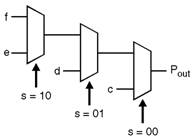Home > VHDL > Logic Circuits > 4 2 Priority Encoders

Priority Encoders using If-Then-Else Statement :

If-then-else statement is mainly used to for sequential statements based on a value. The condition of the if-then-else statement is checked in order against that value until a true condition is found. Figure belo shows the Priority Encoder using If-Then-Else Statement.library IEEE;

use IEEE.std_logic_1164.all;

entity my_if is

port (c, d, e, f : in std_logic;

s : in std_logic_vector(1 downto 0);

pout : out std_logic);

end my_if;

architecture my_arc of my_if is

begin

myif_pro: process (s, c, d, e, f) begin

if s = "00" then

pout <= c;

elsif s = "01" then

pout <= d;

elsif s = "10" then

pout <= e;

else pout <= f;

end if ;

end process myif_pro;

end my_arc;